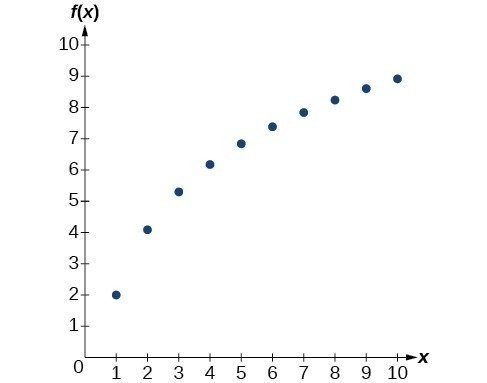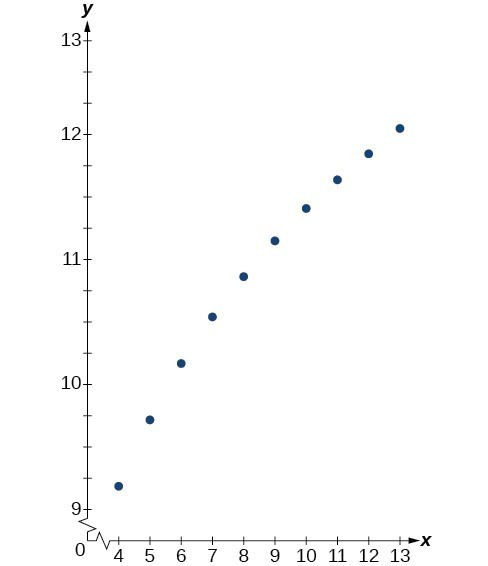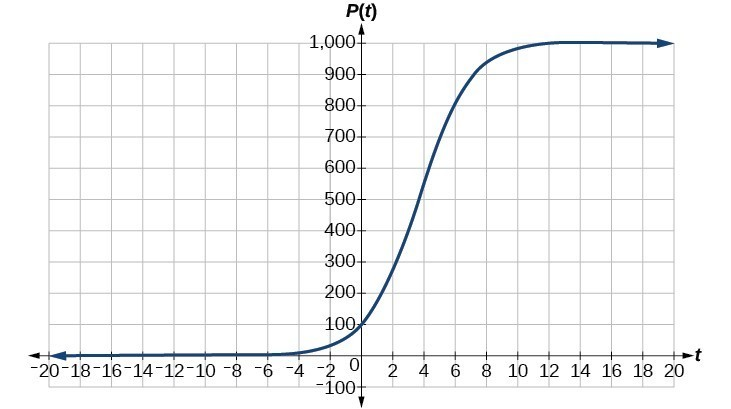Solutions to Try Its

1. $f\left(t\right)={A}_{0}{e}^{-0.0000000087t}$

2. less than 230 years, 229.3157 to be exact

3. $f\left(t\right)={A}_{0}{e}^{\frac{\mathrm{ln}2}{3}t}$

4. 6.026 hours

5. 895 cases on day 15

6. Exponential. $y=2{e}^{0.5x}$.

7. $y=3{e}^{\left(\mathrm{ln}0.5\right)x}$

Solutions to Odd-Numbered Exercises

1. Half-life is a measure of decay and is thus associated with exponential decay models. The half-life of a substance or quantity is the amount of time it takes for half of the initial amount of that substance or quantity to decay.

3. Doubling time is a measure of growth and is thus associated with exponential growth models. The doubling time of a substance or quantity is the amount of time it takes for the initial amount of that substance or quantity to double in size.

5. An order of magnitude is the nearest power of ten by which a quantity exponentially grows. It is also an approximate position on a logarithmic scale; Sample response: Orders of magnitude are useful when making comparisons between numbers that differ by a great amount. For example, the mass of Saturn is 95 times greater than the mass of Earth. This is the same as saying that the mass of Saturn is about ${10}^{\text{2}}$ times, or 2 orders of magnitude greater, than the mass of Earth.

7. $f\left(0\right)\approx 16.7$; The amount initially present is about 16.7 units.

9. 150

11. exponential; $f\left(x\right)={1.2}^{x}$

13. logarithmic15. logarithmic17.23. 4 half-lives; 8.18 minutes

25. $\begin{cases}\text{ }M=\frac{2}{3}\mathrm{log}\left(\frac{S}{{S}_{0}}\right)\hfill \\ \mathrm{log}\left(\frac{S}{{S}_{0}}\right)=\frac{3}{2}M\hfill \\ \text{ }\frac{S}{{S}_{0}}={10}^{\frac{3M}{2}}\hfill \\ \text{ }S={S}_{0}{10}^{\frac{3M}{2}}\hfill \end{cases}//$

27. Let $y={b}^{x}$ for some non-negative real number b such that $b\ne 1$. Then,
$\begin{cases}\mathrm{ln}\left(y\right)=\mathrm{ln}\left({b}^{x}\right)\hfill \\ \mathrm{ln}\left(y\right)=x\mathrm{ln}\left(b\right)\hfill \\ {e}^{\mathrm{ln}\left(y\right)}={e}^{x\mathrm{ln}\left(b\right)}\hfill \\ y={e}^{x\mathrm{ln}\left(b\right)}\hfill \end{cases}$

29. $A=125{e}^{\left(-0.3567t\right)};A\approx 43$ mg

33. $f\left(t\right)=250{e}^{\left(-0.00914t\right)}$; half-life: about $\text{76}$ minutes

35. $r\approx -0.0667$, So the hourly decay rate is about 6.67%

37. $f\left(t\right)=1350{e}^{\left(0.03466t\right)}$; after 3 hours: $P\left(180\right)\approx 691,200$

39. $f\left(t\right)=256{e}^{\left(0.068110t\right)}$; doubling time: about 10 minutes

43. $T\left(t\right)=90{e}^{\left(-0.008377t\right)}+75$, where t is in minutes.
47. $\mathrm{log}\left(x\right)=1.5;x\approx 31.623$
51. $N\left(3\right)\approx 71$